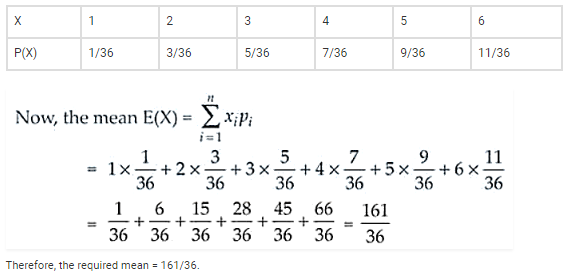Deepak Scored 45->99%ile with Bounce Back Crack Course. You can do it too!

# Find the probability distribution of the maximum

Question:

Find the probability distribution of the maximum of the two scores obtained when a die is thrown twice. Determine also the mean of the distribution.

Solution:

Let X be the random variable scores when a die is thrown twice.

X = 1, 2, 3, 4, 5, 6

And S = {(1, 1), (1, 2), (2, 1), (2, 2) , (1, 3) , (2, 3) , (3, 1) , (3, 2) , (3, 3) , (3, 4) , (3, 5) , …, (6, 6)}

So,

P(X = 1) = 1/6 . 1/6 = 1/36

P(X = 2) = 1/6 . 1/6 + 1/6 . 1/6 + 1/6 . 1/6 = 3/36

P(X = 3) = 1/6 . 1/6 + 1/6 . 1/6 + 1/6 . 1/6 + 1/6 . 1/6 + 1/6 . 1/6 = 5/36

Similarly,

P(X = 4) = 7/36; P(X = 5) = 9/36 and P(X = 6) = 11/36

Hence, the required distribution is